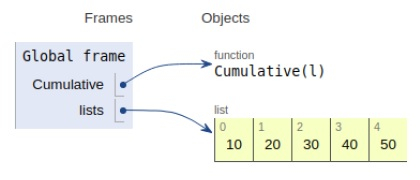# Python program to find Cumulative sum of a list

Problem statement − We are given a list, we need to create a list with the cumulative sum.

Now let’s observe the solution in the implementation below −

## Example

Live Demo

# cumulative sum
def Cumulative(l):
new = []
cumsum = 0
for element in l:
cumsum += element
new.append(cumsum)
return new
# Driver Code
lists = [10, 20, 30, 40, 50]
print ("New list:",Cumulative(lists))

## Output

New list: [10, 30, 60, 100, 150]All the variables are declared in the local scope and their references are seen in the figure above.

## Conclusion

In this article, we have learned about how we can make a Python program to find the Cumulative sum of a list.